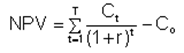# Net Present Value

Financial analysis Print Email

As explained by financial authors, the Net Present Value or Net Present Worth is defined as the present values of the individual cash flows, both incoming and outgoing, of a business entity. Net Present Value (NPV) is, basically, used in capital budgeting so as to study the profitability resulting from a project or an investment. However, the analysis of NPV is susceptible to the reliability of future cash inflows that will be yielded by a project or investment plan.

Calculation (formula)T – number of years,
Ct –proceeds (incoming cash flow) for the year t,
Co – initial expenses (outgoing cash flow) .

In addition to the above mentioned formula, the NPV can also be calculated with the help of tables and spreadsheets like MS Excel.

Any prospective project would be considered acceptable if it features a positive NPV and the same can also be rejected if it contains a negative NPV. For instance, let’s take the case of a retail clothing business’ desire to buy an already existing store. As a part of the process the buyer would first analyze the future cash flow that would be generated by the store. Thereafter, these cash flows are discounted into a total present value amount, say \$655,000. If, in case, the owner of the store is ready to sell his business for a lesser amount, the buyer would probably accept the offer for it reflects a positive NPV investment.

On the contrary, if the owner of the store is not willing to sell it for a lesser amount, the buyer would, probably, not buy the store for this investment might lead to a negative NPV thereby leading to a downfall in the overall company value.

Adding more to the point, the calculation of NPV is sensitive to the discount rate. Even the smallest of change in the discount rates can lead to large changes in the Net Present Value (NPV). Thus, the Net Present Value numbers are quite uncertain due to the uncertain nature of the discount rates. Besides, a Net Present Value also, sometimes, depends upon uncertain forecasts about the prospective cash flows thereby creating a problem in the calculation of the Net Present Value.

Well, as a solution to both these problems, a range of discount rates and forecasts can be used to calculate a range of Net Present Value thereby generating the best, worst, and the median case NPV numbers or a probability distribution for the Net Present Value.